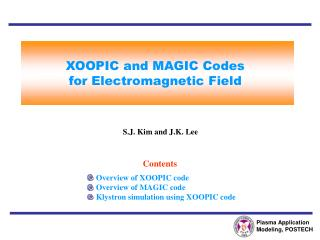DownloadDownload PresentationPlasma Application Modeling, POSTECH

# Plasma Application Modeling, POSTECH

Télécharger la présentation## Plasma Application Modeling, POSTECH

- - - - - - - - - - - - - - - - - - - - - - - - - - - E N D - - - - - - - - - - - - - - - - - - - - - - - - - - -
##### Presentation Transcript

1. Plasma Application Modeling, POSTECH XOOPIC and MAGIC Codes for Electromagnetic Field S.J. Kim and J.K. Lee Contents • Overview of XOOPIC code • Overview of MAGIC code • Klystron simulation using XOOPIC code

2. Plasma Application Modeling, POSTECH Overview of XOOPIC Code XOOPIC Features • Two dimension and three velocity • Cartesian (x-y) or cylindrical (r-z) • coordinates • Electrostatic or full electromagnetic field • Discrete model (Finite-Difference Method) • : uniform or non-uniform mesh • Boltzmann and inertial electrons • Immobile and inertial ions • Monte-Carlo collision model • Complex boundaries : conductor, cylindrical axis, wave ports, • absorption, transmission, emission. * Values of gridded quantities can be approximated at intermediate points by interpolation.

3. Plasma Application Modeling, POSTECH Program Flow Electromagnetic fields on the mesh Discretization mesh Defined region of the discrete model Individual particle (position, momentum, mass, charging, numerical weight) Group of similar particles

4. Plasma Application Modeling, POSTECH Maxwell’s Equations for Electromagnetic Field Maxwell’s equations in integral form C-1, L-1: coupling matrices with the dimemsionality of capacitance and inductance. Nonuniform orthogonal Yee mesh

5. Plasma Application Modeling, POSTECH Maxwell Curl Equations Transverse magnetic (TM) set Transverse electric (TE) set The TM and TE field equations are advanced in time using a leap frog advance. The currents result from charged particle motion.

6. Plasma Application Modeling, POSTECH Velocity Advance Relativistic Boris advance • Half acceleration: • Rotation: • Half acceleration:

7. Plasma Application Modeling, POSTECH Charge Conserving Current Weighting Algorithm Charge conserving current weighting =

8. Plasma Application Modeling, POSTECH Overview of MAGIC Code MAGIC code Grid Materials Resistive Dielectric Conductors Scattering foil Polarizer sheet Helix element General current source Air chemistry Semiconductor • Particle-in-cell (PIC) approach • Maxwell’s equations on a finite-difference • grid for electromagnetic field Uniform grid Manual grid Appended regions Polynomial smoothly varying grid Pade smoothly varying grid Electromagnetic computational processing cycle Geometry Field algorithm Cartesian coordinates Polar coordinates Cylindrical coordinates Spherical coordinates Mirror symmetry boundary Periodic symmetry boundary Absorbing boundary Outgoing wave boundary Applied voltage boundary External circuit voltage source Particle and field import Standard leapfrog Time-reversible leapfrog Semi-implicit Standard noise filtering High-Q noise filtering Quasistatic Electrostatic ADI Electrostatic SOR Externally specified magnet field Restricted TE or TM modes • Application fields : microwave amplifiers, • antennas, sensors, fiber optics, lasers, • accelerator components, beam propagation, • pulsed power, plasma switches, microwave • plasma heating, ion sources, field emitter • arrays, semiconductor devices, wave • scattering, and coupling analyses

9. Plasma Application Modeling, POSTECH Method and Noise Leapfrog time integration scheme Well-centering • Particle-induced noise is introduced through the current term in Maxwell’s equations. Transverse particle noise Longitudinal particle noise • Spatial fluctuations in space charge and • the Gauss’s law constraint • The slow, self-heating instability • Charge allocation algorithm • Propagating, wave-like, electromagnetic nose • Large curl derivatives • Time-biased and high-Q algorithms

10. Plasma Application Modeling, POSTECH Time-Biased Algorithm Time-biased algorithm : semi-implicit scheme a1, a2, and a3 determine the degree of spatial filtering and the time-centering. i : iteration coefficient =k/kmax : normalized eigenmode kmax : maximum spatially-resolvable Fourier wave number

11. Charge Conservation Scheme Langdon-Marder correction Boris-DADI correction

12. Plasma Application Modeling, POSTECH Simulation Domain of Klystron RF output port RF input port 9.55 cm 10.05 cm 13.07 cm E-beam 7.569 cm 6.66 cm Cylindrical Axis 37.2 cm • Simulation condition: • Beam emitter: I= 12 kA, ud =2.48e8 m/s • Input port : Rin=2300 , R=20 , f=7.69 GHz • Output port : R=47.124 

13. Plasma Application Modeling, POSTECH Example of Klystron Simulation Phase space Density uz Kinetic energy

14. Plasma Application Modeling, POSTECH Simulation Results at 0.5 ns

15. Plasma Application Modeling, POSTECH Simulation Results at 2.5 ns

16. Plasma Application Modeling, POSTECH Simulation Results at 10 ns

17. Plasma Application Modeling, POSTECH Simulation Results at 20 ns

18. Plasma Application Modeling, POSTECH Simulation Results at 6 us

19. Plasma Application Modeling, POSTECH KE as a Function of Beam Current

20. Plasma Application Modeling, POSTECH KE as a Function of Beam Energy

21. Plasma Application Modeling, POSTECH Klystron 3 cm 2 cm Phase space Density uz Kinetic energy

22. Plasma Application Modeling, POSTECH Simulation Results at 0.5 ns and 2.5 ns

23. Plasma Application Modeling, POSTECH Simulation Results at 10 ns and 20 ns

24. Plasma Application Modeling, POSTECH Simulation Results at 6 us